Courses

# Notes | EduRev

## Mathematics (Maths) Class 10

Created by: Indu Gupta

## Class 10 : Notes | EduRev

The document Notes | EduRev is a part of the Class 10 Course Mathematics (Maths) Class 10.
All you need of Class 10 at this link: Class 10

Class X
Mathematics – Standard (041)
Sample Question Paper 2019-20

Max. Marks: 80
Duration : 3 hrs

General Instructions:
(i) All the questions are compulsory.
(ii) The question paper consists of 40 questions divided into 4 sections A, B, C, and D.
(iii) Section A comprises of 20 questions of 1 mark each. Section B comprises of 6 questions of 2 marks each. Section C comprises of 8 questions of 3 marks each. Section D comprises of 6 questions of 4 marks each.
(iv) There is no overall choice. However, an internal choice has been provided in two questions of 1 mark each, two questions of 2 marks each, three questions of 3 marks each, and three questions of 4 marks each. You have to attempt only one of the alternatives in all such questions.
(v) Use of calculators is not permitted.

Section A

Q.1. If an event that cannot occur, then its probability is :    (1 Mark)
(a) 1
(b) 3/4
(c) 1/2
(d) 0
Ans.(d)
An event that cannot occur has 0 probability, such an event is called impossible event.

Q.2. The smallest rational number by which 1/7 should be multiplied so that its decimal expansion terminates after one place of decimal is:    (1 Mark)
(a) 7
(b) 7/10
(c) 7/100
(d) 1/10

Ans. (b)
[Here, 1/7 is non - terminating decimal expansion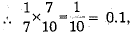which terminates after one place of decimal]

Q.3. The distance between two parallel tangents to a circle of radius 7 cm, is    (1 Mark)
(a) 49 cm
(b) 7 cm
(c) 14 cm
(d) 28 cm
Ans. (c) The distance between two parallel tangents to a circle of radius 7 cm is 2 x 7 = 1 4 qm.

Q.4. The zeroes of the quadratic polynomial x2 - 13x + 40 are    (1 Mark)
(a) 8, 5
(b) - 8, - 5
(c) 8, -5
(d) -8, 5
Ans.
(a)

The zeroes of the given polynomial are given by the equation
x2 - 13x + 40 = 0
⇒ x2 - 5x - 8x + 40 - 0
⇒ x(x - 5) - 8(x - 5) = 0
⇒ (x - 5)(x - 8) = 0
⇒ x = 5 = 0 or x - 8 = 0
⇒ x = 5 or x = 8
Hence, the zeroes of the Polynomial are 5 and 8.

Q.5. (sec A + tan A) (1 - sin A) =    (1 Mark)
(a) sec A
(b) sin A
(c) cosec A
(d) cos A
Ans. (d)
(sec A + tan A) (1 - sin A)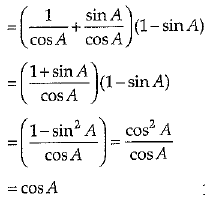Q.6. If p sin (90° - θ) cot (90° - θ) = cos (90° - θ), then x =    (1 Mark)
(a) 0
(b) 1
(c) - 1
(d) 2
Ans. (b)
[Here, p sin (90° - θ) cot (90° - θ)
= cos (90° - θ) ⇒ cos θ.tan θ = sin θ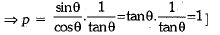Q.7. Which of the following is a solution of 2x2 - 5x - 3 = 0 ?    (1 Mark)
(a) x = 0
(b) x = 1
(c) x = 3
(d) x = 2
Ans.  (c) Given, 2x2 - 5x - 3 = 0
Splitting the middle term we get,
2x2 - 6x+ x - 3 = 0
⇒ 2x (x - 3) + 1 (x - 3) = 0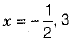Hence, x = 3 is the solution.

Q.8. The probability of a sure event is __________.    (1 Mark)
Ans. 1

Q.9. If the zeroes of the quadratic polynomial ax2 + bx + c, a ≠ 0 are equal, then :    (1 Mark)
(a) c and a have opposite signs
(b) c and b have opposite signs
(c) c and a have the same sign
(d) c and b have the same sign
Ans. : For equal roots b2 - 4ac = 0 or b2 = 4ac
b2 is always positive so 4ac must be positive , i.e., product of a and c must be positive, i.e., a and c must have same sign either positive or negative.

Q.10. The equation of the perpendicular bisector of AB, where A and B are the points (3, 6) and (- 3, 4) is :    (1 Mark)
(a) 3x + y - 5 = 0
(b) x + 3y - 5 = 0
(c) x - 3y + 5 = 0
(d) - x - 3y + 5 = 0
Ans. (a) 3x + y - 5 = 0
[Here, PA = PB, where P(x, y) is any point on the perpendicular bisector of AB.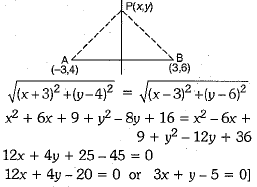Q.11. Suppose 0 < θ, φ < 90° and sin θ and cos φ will be equal, when θ and φ both are equals to......    (1 Mark)
Ans. (π/4) sin θ and cos will be equal, when
θ = φ = π/4

Q.12. The probability of face card of spade from a pack of cards is 3/52.    (1 Mark)
Ans. True
Total number of outcomes = 52
Number of favourable events = 3 as there are only 3 face cards of spade i.e., J, Q, K.
∴ P(Face cards of space) = 3/52.

Q.13. If one root of 7x2 + 16x + k = 0 is the reciprocal of the other root then find value of k.    (1 Mark)
Ans. Let α and 1/α be the roots of the given quadratic polynomial.
∴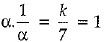⇒ k = 7

Q.14. If 2x, x + 10 and 3x + 2 are in AP, then x = ..........    (1 Mark)
Ans. [x + 10 - 2x = 3x + 2 - (x + 10)
10 - x = 3x + 2 - x - 10
⇒ 3x = 18 ⇒ x = 6]

Q.15. The arithmetic mean between two numbers a and b is .......    (1 Mark)
Ans. AM between a and b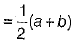Q.16. Find the value(s) of k for which the quadratic equation 3x2 + kx + 3 = 0 has real and equal roots.    (1 Mark)
Ans. The given equation is 3x2 + kx + 3 = 0
Comparing it with the standard equation ax2 + bx + c, we have
a = 3, b = k, c = 3
The roots will be real and equal if discriminant,
D = b2 - 4ac = 0
i.e., k2 - 4 x 3 x 3 = 0
⇒ k2 = 36
⇒ k = ± 6
Hence, the roots of the equation are real and equal for k = ± 6.

Q.17. Find the distance of the point (- 4, - 7) from the y-axis.    (1 Mark)
Ans. Points are (- 4, - 7) and (0, - 7)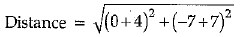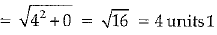Q.18. Find the sum of all numbers between 250 and 1000 which are exactly divisible by 3.    (1 Mark)
Ans. Numbers between 250 and 1000, which are exactly divisible by 3 are :
252, 255, 258, ..., 999
Using a + (n - 1)d = an, we have
252 + (n - 1)3 = 999
(n - 1)3 = 999 - 252
= 747
= n - 1 = 747/3 = 249
∴ n = 249 + 1 = 250
Now, required sum of all such numbers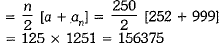Q.19. Find the nature of the roots of the equation 3x2 +6a + 1 = 0.
Or
Find the values of t, so that (t + 4)x2 + (t + 1)x +1 = 0 has real and equal roots.    (1 Mark)

Ans. Given, 3a+ 6x + 1 = 0
a = 3,b = 6 andc = 1
Discriminant, D = b2 - 4ac
= (6)2 - 4 x 3 x 1
= 36 - 12 = 24 >0
Hence, the given equation has two distinct real roots.
Or
Discriminant = b2 - 4ac
= (t + 1)2 - 4(t + 4) x 1
= t2 - 2t - 15
For equal roots, discriminant = 0
∴ t2 - 2t - 15 = 0
⇒ t2 - 5t + 3t - 15 = 0
⇒ t = 5 or t = - 3
Hence, the value of t is 5, - 3.

Q.20. Find the value of ‘a’ so that the point (3, a) lies on the line represented by 2x - 3y = 5.    (1 Mark)
Ans. The point (3, a) lies on the line given by the equation 2x - 3y = 5
The coordinates of the point satisfy the above equation.
2 x 3 - 3 x a = 5
⇒ - 3a = - 1
⇒ a = 1/3

Section B

Q.21. Explain whether 3 x 12 x 101 + 4 is a prime number or a composite number.    (2 Mark)
Ans. 3 x 12 x 101 + 4= 4(3 x 3 x 101 + 1)
= 4(909 + 1) ................
= 4(910)
= 2 x 2 x 2 x 5 x 7 x 13
= a composite number
[∵ Product of more than two prime factors]

Q.22. Prove that the segment joining the points of contact of two parallel tangents passes through the centre.    (2 Mark)
Ans. Let P be the point of contact and l be the tangent at the point P on the circle with centre O.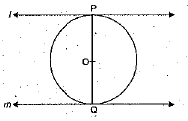Since OP is the radius of the circle and 1 is tangent.
∴ OP ⊥ l
Similarly, OQ ⊥ m
Since l and m are two parallel tangents.
∴ Perpendicular at P and Q passes through the centre of the circle.

Q.23. A teacher of Class X write some information about the tour on blackboard as follows:
Beginning at 8:30 am, tours of the national capital and the white house begin at a tour agency. Tours for the national capital leave every 15 min. Tours for the white house leave every 20 min. How many minutes after do the tours leave at the same time ?    (2 Mark)
Ans. Required time = LCM (15, 20).
By using prime factorisation method,
Now, 15 = 3 x 5
and 20 = 2 x 2 x 5 = 2 2 x 5
∴ LCM (15, 20) = 22 x 3 x 5 = 60 min
In every 60 min, tour leaves at the same time.

Q.24. Find the HCF of 612 and 1314 using prime factorisation.
Or
Show that any positive odd integer is of the form 6m + 1 or 6m + 3 or 6m + 5, where m is some integer    (2 Mark)
Ans. We have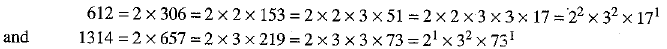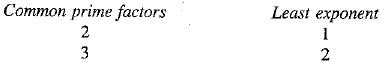∴ HCF = 21 x 32 = 2 x 9 = 18
Or
Let a be any odd positive integer.
When a is divided by 6, remainder will be one of 0, 1, 2, 3, 4, 5.
a = 6m + 0 i.e., 6m or 6m +1 or 6m + 2 or 6m + 3 or 6m + 4 or 6m + 5
When a = 6m, which is even positive integer as it is divisible by 2.
When a = 6m + 2 = 2(3m + 1), which is even positive integer as it is divisible by 2.
When a = 6m + 4 = 2(3m + 2), which is even positive integer as it is divisible by 2.
Hence, every odd positive integer is of the form 6m + 1 or 6m + 3 or 6m + 5.

Q.25. Find the ratio in which y-axis divides the line segment joining the points A(5, -6) and B(-1, -4). Also find the co-ordinates of the point of division.    (2 Mark)
Ans. Let the point on y-axis be (0, y) and AP :PB = k: 1.
Therefor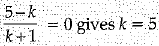Hence, required ratio is 5 :1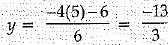Hence, point on y- axis is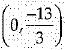Q.26. Due to heavy floods in a state, thousands were rendered homeless. 50 schools collectively offered to the state government to provide place and the canvas for 1500 tents to be fixed by the government and decided to share the whole expenditure equally. The lower part of each tent is cylindrical of base radius 2.8 m and height 3.5 m, with conical upper part of same base radius but of height 2.1 m. If the canvas used to make the tents cost Rs. 120 per sq. m, find the amount shared by each school to set-up the tents.[Use π = 22/7]    (2 Mark)
Ans. Here, radius of base of cylindrical portion = radius of base of conical portion (r)
= 2.8 m
Height of cylindrical portion = 3,5 m
Height of conical portion = 2.1 m
∴ Slant height of conical portion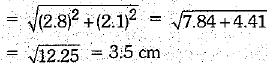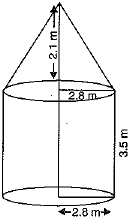Area of canvas used to make the tent
=C.S.A. of cylindrical portion + C.S.A. of conical portion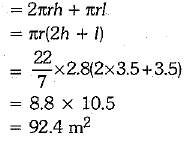Section C

Q.27. Find the roots of the quadratic equation :    (3 Mark)
a2b2x2 + b2x - a2x - 1 = 0
Ans. Given, a2b2x2 + b2x - a2x - 1 = 0
⇒ b2x(a2x + 1) - 1(a2x + 1) = 0
⇒ (b2x - 1)(a2x + 1) = 0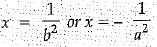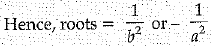Q.28. A spiral is made-up of successive semicircles, with centres alternately at A and B, starting with centre at A, of radii 0.5 cm, 1.0 cm, 1.5 cm, 2 .0 c m , ... as shown in figure. What is the total length of such a spiral made-up of 13 consecutive semicircles ?    (3 Mark)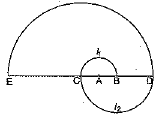Ans. Since radii of semicircles are 0.5 cm, 1.0 cm, 1.5 cm, 2.0 cm ...
Now, length of first semicircle (l1)
= Perimeter of first semicircle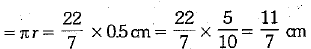Now, length of second semicircle (l2)
= Perimeter of second semicircle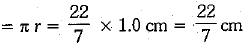Similarly, we can get the length of other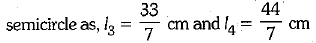and so on upto 13 semicircles.
Let S be total length of all semicircles.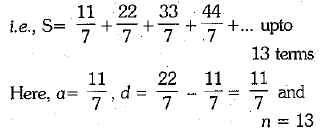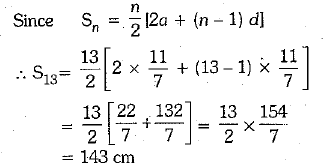Q.29. Find the value of p for which the points A (- 1, 3), B (2, p) and C (5, -1) are collinear. Also, find the ratio in which B divides AC.
Or
The two opposite vertices of a square are (-1,2) and (3,2). Find the coordinates of the other two vertices.    (3 Mark)
Ans. If the given points A (-1, 3), B(2, p) and C(5, -1) are coilinear, then area of the triangle formed by these points is 0.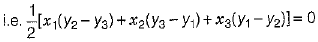⇒ x1(y2 - y3)+x2(y3 - y1) + x3(y1 - y2) = 0
⇒ -1(p + 1)+2(-1-3) + 5(3-p) = 0
⇒ - p - 1 - 8 + 15 - 5p = 0
⇒ - 6p + 6 = 0 ⇒ p = 1
Thus, for p = 1, the given points are coliinear.
Now, let S(2,p) = B(2,1) divides the line segment joining the points A and C internally in the ratio k:1.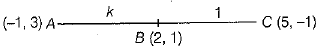By using internal section formula, we get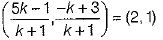⇒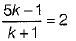[comparing x-coordinate from both sides]
⇒ 5k - 1 = 2k + 2
⇒ 5k - 2k = 2 + 1
⇒ 3k = 3 ⇒ k = 1
Hence, the required ratio is 1:1.
Or
Let PQRS be a square with opposite vertices P (- 1,2) and R (3,2). Again, iet the coordinates of other vertices Q be (x, y).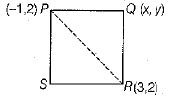∵ All sides of a square’are equal,
∴ PQ = QR
⇒ PQ2 = QR2 [squaring both sides]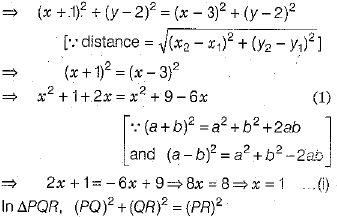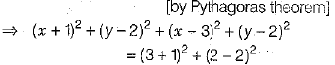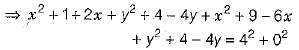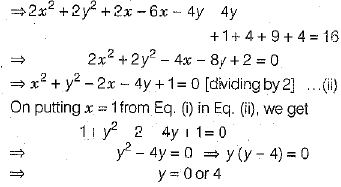Hence, other two vertices of the square are (1,0) and (1, 4).

Q.30. A part of monthly hostel charges in a college hostel are fixed and the remaining depends on the number of days one has taken food in the mess. When a student A takes food for 25 days, he has to pay Rs. 4,500, whereas a student B who takes food for 30 days, has to pay Rs. 5,200. Find the fixed charges per month and the cost of food per day.    (3 Mark)
Ans. Let the fixed hostel charges be Rs. x and the cost of food per day be Rs. y.
∴ Total charges for 25 days = Fixed hostel charges + Food charges for 25 days
= Rs. (x + 25y) = Rs. 4500 (Given)
∴  x+ 25y = 4500 ...(1)
Similarly, x + 30y = 5200    ...(2)
Subtracting (1) from (2), we get
5y = 70
⇒ y = 140
Putting the value of y from (3) in (1), we get     ...(3)
x + 25 x 140 = 4500
x + 3500 = 4500
x = 4500 - 3500 = 1000
∴ Fixed hostel charges = Rs. 11000 and cost of food per day = Rs. 140.

Q.31. The points A(1, - 2), B(2,3), C(k, 2) and D(- 4, - 3) are the vertices of a parallelogram. Find the value of k.    (3 Mark)
Ans.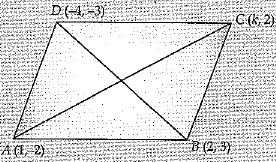Diagonals of parallelogram bisect each other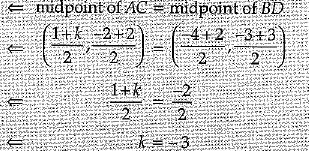Q.32. If tan A = 2, evaluate : sec A sin A + tan2A - cosec A.
Or
If A + B = 90°, then prove that:    (3 Mark)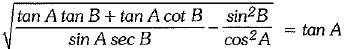Ans. We know that, cosec2A = 1 + cot2A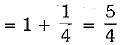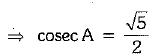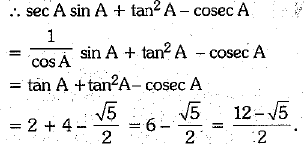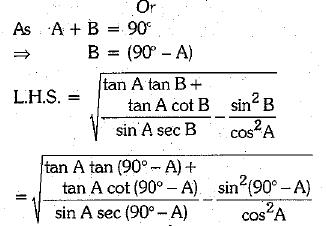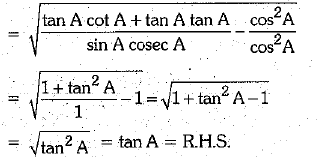Q.33. In the figure, ABC is a quadrant of a circle of radius 14 cm and a semi-circle is drawn with AC as a diameter. Find the area of the shaded region.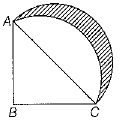Or
A horse is tied to a peg at one corner of a square shaped grass field of side 15 m by means of a 5 m long rope (see the figure).    (3 Mark)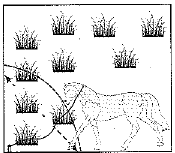Find
(i) the area of the part of the field in which the horse can graze.
(ii) the increase in the grazing area if the rope were 10m long instead of 5 m.[take, π = 3.14]    (3 Mark)
Ans. In right angled ΔABC,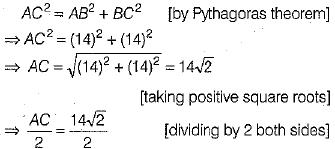∴ Radius of semi-circle, ACQA = 7√2 cm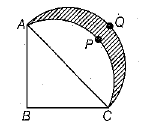Now, area of shaded portion = Area of APCQA
= Area of semi-circle ACQA - (Area of quadrant BAPC - Area of ΔABC)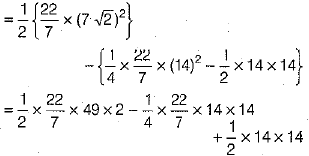= 154 - 154 + 98
= 98 cm2
Or
Given, length of rope = 5 m
Radius of arc = 5 m
(i) Area of the field the horse can graze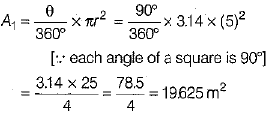(ii) If length of rope = 10 m = r1 (say)
Then, area of the field graze by the horse,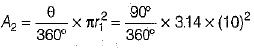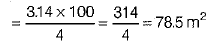∴ Required increase in the grazing area
= A2 - A1 = 78.5 m2 -  19.625 m2
= 58.875 m2

Q.34. A girl empties, a cylindrical bucket full of sand, of base radius 18 cm and height 32 cm on the floor to form a conical heap of sand. If the height of this conical heap is 24 cm, then find its slant height correct to one place of decimal.    (3 Mark)
Ans. Radius of cylindrical bucket, r = 18 cm and height of cylindrical bucket, h = 32 cm
∴ Volume of the sand in the cylindrical bucket = πr2h = (18)2 x 32π cm3 = (18 x 18 x 32)π cm3
Height of conical heap, H = 24 cm
Let R be the radius of the conical heap.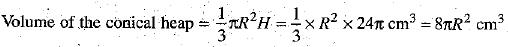Volume of the cylindrical bucket = Volume of the conical heap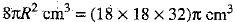⇒ R2 = (18 x 18 x 4)
⇒ R = 36 cm
Slant height l of the conical heap is given by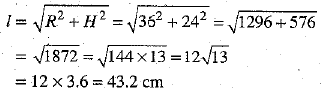Hence, the slant height of the heap = 43.2 cm

Section D

Q.35. The following table gives the weight of 120 articles :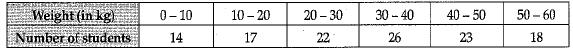Change the distribution to a 'more than type' distribution and draw its give.    (4 Mark)
Ans.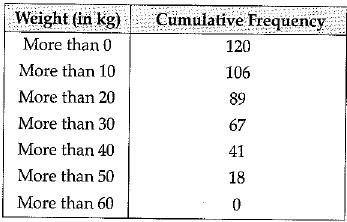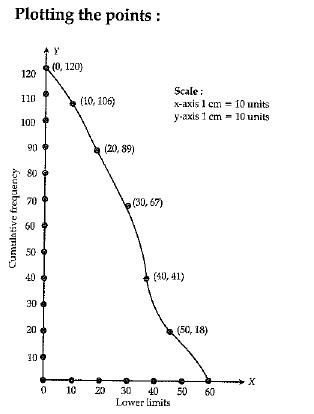Q.36. In the given figure, the line segment PQ is parallel to AC of ΔABC and it divides the triangle into two parts of equal area. Find the ratio AP/AB.    (4 Mark)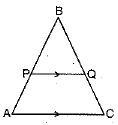Ans. In ΔABC, PQ || AC
∠A = ∠P and ∠C = ∠Q
In ΔBPQ and ΔBAC [∵ corr. angles]
∠B = ∠B [common]
∴ ΔBPQ ~ ΔBAC
[by AAA similarity rule]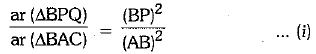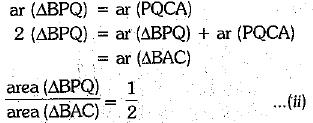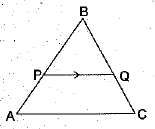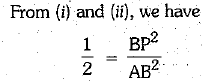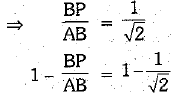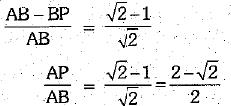Q.37. Prove that the line segments joining the mid-points of the sides of a triangle form four triangles, each of which is similar to the original triangle.
Or
In ΔPQR, PS⊥ R and (PS)2 = QS x RS.
Prove that ΔPQR is a right angled triangle.    (4 Mark)
Ans. Let D, E and F are the mid-points of sides BC, CA and AB respectively of ΔABC.DE, EF and FD are joined.
To prove ΔAFE, ΔFBD, ΔEDC and ΔDEF are similar to ΔABC.
Proof In ΔABC, F and E are the mid-points of sides AB and AC, respectively
FE ║ BC
[segment joining the mid-points of any two sides of triangles is parallel to the third side]
Similarly, DF ║ CA and DE ║ BA
In ΔAFE and ΔABC,
∠A = ∠A [common angle]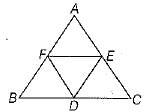∠AFE = ∠B [corresponding angles]
∴  ΔAFE ∼ ∠ABC ...(i)
[by AA similarity criterion]
Similarly, we can prove
ΔFBD ∼ ΔABC ...(ii)
and ΔEDC ∼ ΔABC      ...(iii).
Now, in ΔDEF and ΔABC,
∠D = ∠A
[opposite angles of parallelogram AFDE]
∠DEF = ∠B
[opposite angles of parallelogram BDEF]
∴  ΔDEF - ΔABC     ......(iv)
[by AA similarity criterion]
From Eqs. (i), (ii), (iii) and (iv), we conclude that each of ΔAFE, ΔFBD, ΔFDC and ΔDEF is similar to ΔABC. Hence proved.
Or
In right angled ΔPSQ and ΔPSR,
(PQ)2 = (PS)2 + (QS)2 .......(i)
and (PR)2 = (PS)2 + (SR) ...(ii)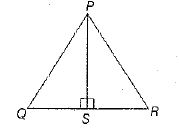On adding Eqs, (i) and (ii), we get (PQ)2 + (PR)2 = 2 (PS)2 + (QS)2 + (SR)2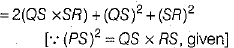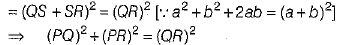Hence, ΔPQR is a right angled triangle, right angled at P.

Q.38. A boy standing on a horizontal plane finds a bird flying at a distance of 100 m from him at an elevation of 30°. A girl standing on the roof of a 20 m high building, finds the elevation of the same bird to be 45°. The boy and the girl are on the opposite sides of the bird. Find the distance of the bird from the girl.    [Given √2 = 1.414]
Or
The angle of elevation of an aeroplane from a point A on the ground is 60°. After a flight of 30 seconds, the angle of elevation changes to 30°. If the plane is flying at a constant height of 3600√3 metres, find the speed of the aeroplane.    (4 Mark)

Ans. Let A be the position of the bird; C be the position of the boy standing on horizontal plane and E be the position of the girl standing on the roof of building of height DE = 20 m. Draw AB ⊥ on horizontal from A and draw EF ⊥ from E on AB. Then,

AC = 100 m, ∠ACB = 30° and ∠AEF = 45°
From right ΔACB, we have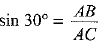⇒ 1/2 = AB/100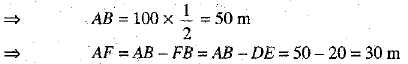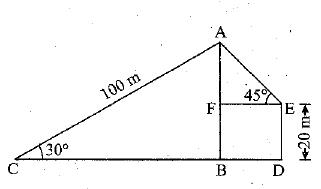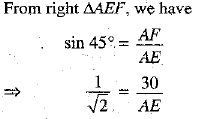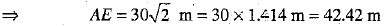Hence, distance of the bird from the girl = 42.42 m.
Or
Let P and Q be initial and final positions of the aeroplane. Let ABC be the horizontal line through point of observation A It is given that the angles of elevation of the plane in two positions P and Q from the point A are 60° and 30° respectively. Draw Pi? and QC perpendiculars from P and Q on the horizontal. The plane is flying at a constant height 3600√3m.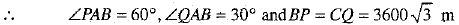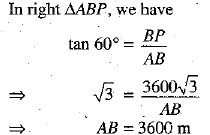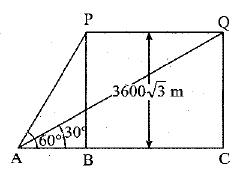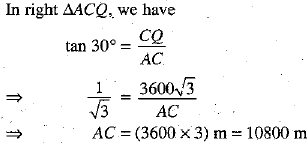Distance travelled by the plane = PQ = BC = AC -AB = (10800- 3600) m = 7200 m
Time taken by the plane in travelling from P to Q = 30 seconds.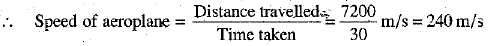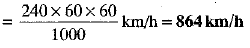Q.39. The numerator of a fraction is 3 less than its denominator. If 2 is added to both the numerator and the denominator, then the sum of the new fraction and original fraction is 29/20. Find the original fraction.    (4 Mark)
Ans. Let the denominator be x, then numerators = x - 3
So, the fraction = x-3/x
By the given condition, new fraction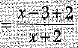= x-1/x+2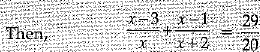⇒ 20[(x-3) (x + 2) + x (x - 1)] - 29(x2 + 2x)
⇒  20(x2 - x - 6 + x2 - x) 29x2 + 58x 1
⇒ 11x2 - 98x - 120 = 0
⇒ 11x2 - 110x + 12x - 120 = 0
(11x + 12) (x - 10) = 0 or x = 10
The fraction is 7/10

Q.40. Find the mean, mode and median for the following data :    (4 Mark)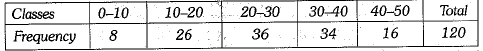Ans.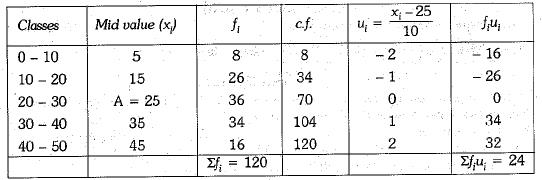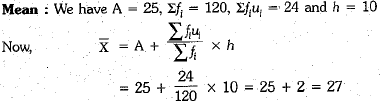Median : We have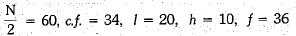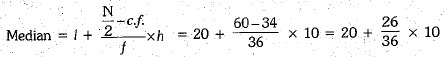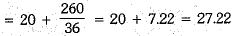Mode : We have
Modal class = 20 - 30 [∵ Max. frequency 36 belongs to 20 - 30]
l = 20, h = 10, f1 = 36, f0 = 26 and f2 = 34
Now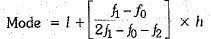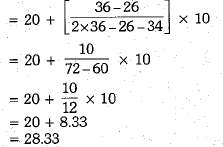Offer running on EduRev: Apply code STAYHOME200 to get INR 200 off on our premium plan EduRev Infinity!

## Mathematics (Maths) Class 10

178 videos|268 docs|103 tests

,

,

,

,

,

,

,

,

,

,

,

,

,

,

,

,

,

,

,

,

,

;